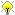[Documentation] [TitleIndex] [WordIndex]Please ask about problems and questions regarding this tutorial on answers.ros.org. Don't forget to include in your question the link to this page, the versions of your OS & ROS, and also add appropriate tags.
Description: Simple Math

Keywords: ecl math

Tutorial Level: BEGINNER

## Macros for PI

Usually there will always be macros for pi defined in <i>math.h</i>, namely M_PI, M_PI_2, etc... (sometimes they're unavailable, e.g. windoze!). For typesafe definitions, the ecl also defines these as constants in the ecl namespace.

```   1 if ( 3.141592653589793238462643383279502884197169399375105820974944 == ecl::pi ) {
2   // ...
3 }
```

## Simple Functions

Various regularly used functions are also present - usually in the form of functors (useful for passing around should it ever be necessary).

Sign

```   1 if ( sign(3) == 1 ) {  // returns -1, 0, 1
2 if ( psign(0) == 1 ) { // returns -1, 1 (0->1)
3
```

Euclidean Norms

```   1 double d1 = EuclideanNorm()(3.0,4.0)) // functor form
2 double d2 = euclidean_norm(3.0,4.0);  // function form
3
```

Cube Root

```   1 double d = ecl::cube_root(8.0); // 2.0
2
```

## Fuzzy Operators

Not, 'fuzzy logic'...

Approximately

```   1 if ( ecl::isApprox(3.0,3.0000000000000001) ) { // ...
2 if ( ecl::isApproxOrLessThan(3.0,3.0000000000000001) ) { // ...
3
```

The bound of the approximation is by default, determined by the machine precision on your machine. If a user specified epsilon bound is preferred, that can be added as an optional third argument to either function.

2020-01-18 12:37